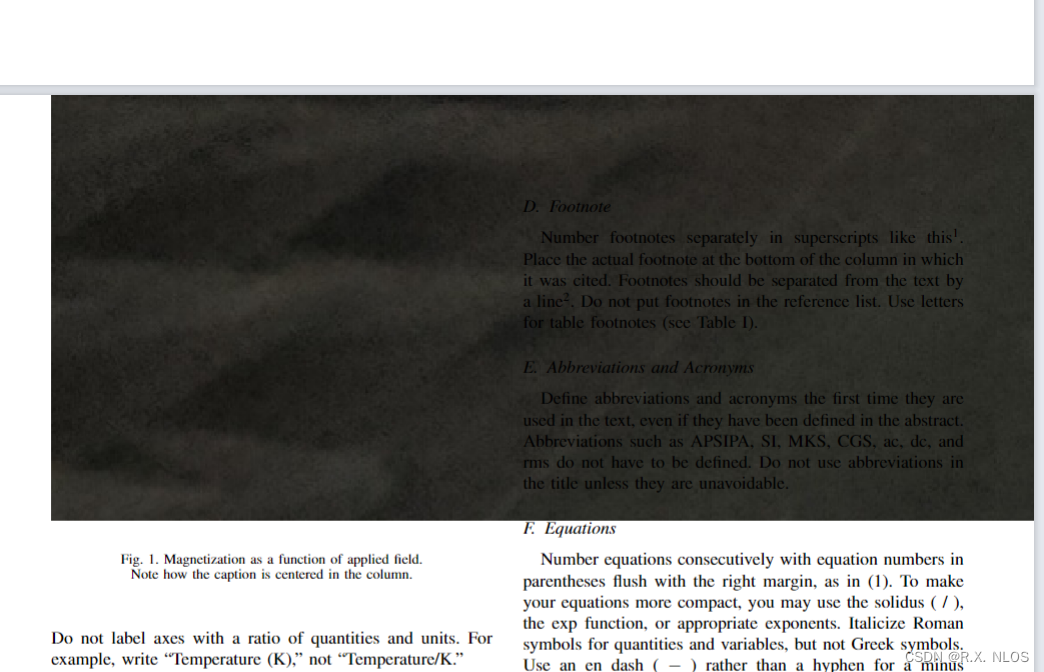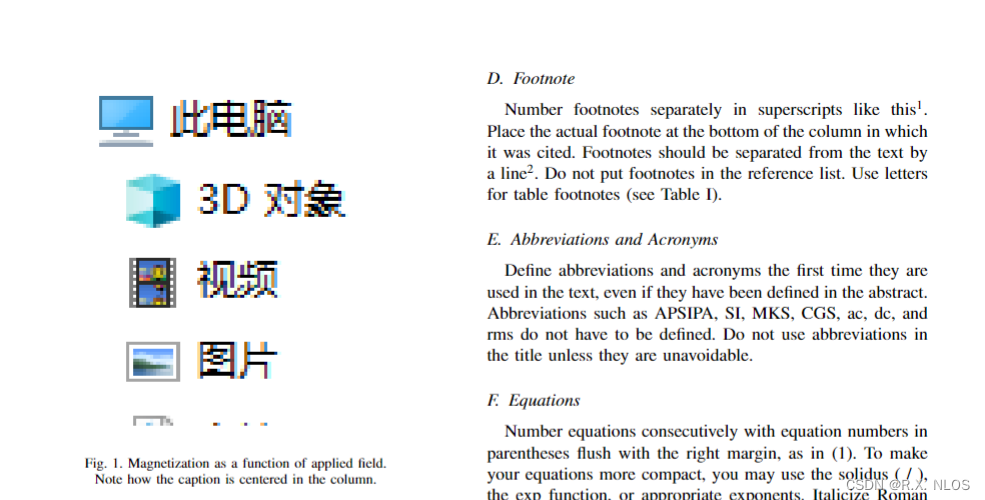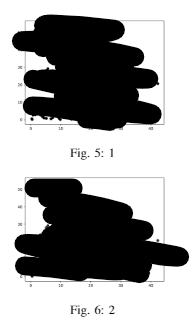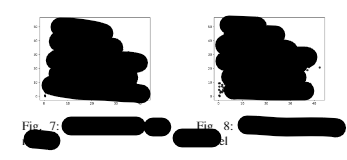• latex双栏排版图片
千次阅读
2022-04-10 10:48:36

Latex 两栏排版中图片、表格需要设置为一栏显示，在{figure}、{table}后加上 *

## 表格操作

\begin{table*}
……
\end{table*}



实例如下：

\begin{table*}[!t]
\centering
...
\label{tab:3}
\caption{……}
\end{table*}



## 图片操作

图片：（占据两栏显示在页面顶端）

\usepackage{stfloats}

\begin{figure*}[ht]
…
\end{figure*}

更多相关内容
• 代码】记录|Latex双栏排版插入图片图片太大的问题一种解决方案。

# 问题描述

使用某会议的latex模板（双栏）完成论文时，出现了插入图片特别大、无法调整大小的问题：

• latex代码
\documentclass[conference,a4paper]{XXX}

...

\usepackage[dvips]{graphicx}

...

\begin{document}

...

\begin{figure}[htbp]
\begin{center}
\includegraphics[width=\linewidth]{Latex/2.jpg}
%\includegraphics[width=\linewidth]{Fig1-TimesNewRomanPSMT.eps}
%\fbox{ \rule[-17mm]{0pt}{30mm} FIGURE }
\end{center}
\caption{Magnetization as a function of applied field.}
\vspace*{-3pt}
{\hfill\footnotesize Note how the caption is centered in the column.\hfill}
\end{figure}

...

\end{document}


• 图片插入结果# 解决方案

• 直接将\usepackage[dvips]{graphicx}修改为\usepackage{graphicx}即可• 原因

• dvips是一个将 DVI 到 PostScript 的驱动程序，适用于latex编译eps图片
• 因此原模版使用latex编译，插入的eps图片是可以正常显示的
• 实际编译的时候默认使用的pdflatex, 所以原模版无法使用，需要将dvips去掉才可以正常编译png、jpg和pdf格式的图片
展开全文• 最近在写论文，整体是双栏排版 需要在单栏插入两个图，并列显示 在网上搜了一圈，主流解决方案如下，参考自知乎 \begin{figure}[htbp] \centering \begin{minipage}[t]{0.48\textwidth} \centering \...

# 问题

最近在写论文，整体是双栏排版
需要在单栏插入两个图，并列显示
在网上搜了一圈，主流解决方案如下，参考自知乎

\begin{figure}[htbp]
\centering
\begin{minipage}[t]{0.48\textwidth}
\centering
\includegraphics[width=5cm]{lsun_ptxt.png} %修改图片名称
\caption{1} % 添加图片描述
\end{minipage}
\begin{minipage}[t]{0.48\textwidth}
\centering
\includegraphics[width=5cm]{lsun_ctxt.png}
\caption{2}
\end{minipage}
\end{figure}


但是最后的效果变成了，单栏双图垂直显示# 解答

我们可以在一个单独figure中，插入两个minipage

\begin{figure}[htbp]
\begin{minipage}[t]{0.45\linewidth}
\includegraphics[width=\linewidth]{figs1.eps} # 修改图片名称
\caption{caption1} # 修改描述
\label{f1}
\end{minipage}%
\hfill%
\begin{minipage}[t]{0.45\linewidth}
\includegraphics[width=\linewidth]{figs2.eps}
\caption{caption2}
\label{f2}
\end{minipage}
\end{figure}


效果如下图，完美解决参考自这里

展开全文• 这里写目录标题）正常插入图片并列插入两张图片 正常插入图片 \begin{figure} \includegraphics[width=0.5\textwidth]{figures/tree.eps} \caption{TGT_GTG​.} \label{tree} \end{figure} 如上述代码所示，首先要有...

# 正常插入图片

\begin{figure}
\includegraphics[width=0.5\textwidth]{figures/tree.eps}
\caption{ T G T_G .} \label{tree}
\end{figure}
如上述代码所示，首先要有正常的begin和end。在第2行中设置图片的大小，并且插入图片的位置和名称，来调用需要插入的图片。第3行代码中，设置图片在论文中的名称，并且为该图片设置标签。设置标签的好处是，在论文中调用该图片时，不需要人为的为每一个图片进行编号，只需要在文章中调用该图片的位置引用该标签，latex就会自动为图片进行编号，并且做到前后对应。（figures/tree.eps表示图片是存储在figures这个文件夹中的，这里写的是图片的存储路径。）
以该图为例，标签是：\label{tree}，使用ref对图片进行调用，在.\后面使用ref{tree}调用，便会在文章中自动对应该图片的编号。这样方便在，如果论文之后需要进行改动，在该图片前面插入了其他图片，该图片的编号发生改变时，论文中对应的编号会自动跟着改变，不需要人为再进行改动。
注：如果需要将图片标题caption居中显示，需要添加一个包：
\usepackage[justification=centering]{caption}

# 并列插入两张图片

\begin{figure}[htbp]
\centering
\begin{minipage}{4cm}
\centering
\includegraphics[width=1\textwidth]{figures/sum.png}
\caption{f u , v _{u, v} ⊕ \oplus f v , w _{v, w} .} \label{sum}
\end{minipage}
\begin{minipage}{4cm}
\centering
\includegraphics[width=1\textwidth]{figures/Min.png}
\caption{Min( f u , v _{u, v} , f v , w _{v, w} ).} \label{min2}
\end{minipage}
\end{figure}
论文中经常会存在这样的情况：两张图片联系很紧密，或者说就是针对同一个例子画了两张图，希望两张图片共用一个图片名称，或者是希望可以并列放在一起，方便查看。
以上述代码为例，首先，正常的begin和end还是要有的，不同的地方是，还需要添加beginminipage和endminipage，这里需要在latex中导入一个新的包：**\usepackage{subfigure}**进行两张图片的并列排列。
其他设置和正常插入图片一样，设置图片大小，插入调用图片的位置和图片名称。设置图片在论文中的名字，设置图片标签。

# 双栏模板中图片通栏并列布局1(不同图片使用不同标题，不同编号)

此例子是针对并列展示的图片拥有各自的标题和编号，例如：与上面图片的并列展示类似，需要使用minipage，不同的地方是：
\begin{figure} 变为 \begin{figure*}
下面举一个例子：
\begin{figure*}
\begin{center}
\begin{minipage}{0.5\textwidth}
\includegraphics[width=1.6in,height=1in]{figures/3edge2.eps}
\caption{example3}
\label{fig:example3}
\end{minipage}
\begin{minipage}{0.5\textwidth}
\includegraphics[width=1.6in,height=1in]{figures/sum.eps}
\caption{example4}
\label{fig:example4}
\end{minipage}
\end{center}
\end{figure*}
以该代码为例，将想要通栏展示的两张图片放在一个\begin{figure*} \end{figure*}中，否则不能并列展示。
\begin{center}是为了是两张并列的图片居中显示在文章中。
\begin{minipage}{0.5\textwidth}中，0.5\textwidth是设置每张图片的宽度，所有图片的宽度之和不能大于1，否则会自动换行，无法并列展示。
\includegraphics[width=1.6in,height=1in]{figures/3edge2.eps}与正常插入图片相同，这一步是设置图片的大小，和设置需要插入图片的位置。
\caption{example3}时设置插入后的图片在文章中的位置。
\label{fig:example3}是设置标签，方便在文章中调用该图片。
如果想要插入不止两张图片，就继续写一个minipage，然后以同样的方式设置图片信息。
如果设置完图片的大小之后发现图片发生变形，可以只设置图片的width，不设置height,这样就会按照图片原比例等比例缩小，不会变形。

# 双栏模板中图片通栏并列布局2（不同图片使有不同的小标题，但拥有一个统一的编号和名称）

此例子是针对并列展示的图片拥有各自的标题，但是编号相同，拥有一个整体的标题，例如：代码示例：
\begin{figure*}
\begin{center}
\subfigure[Vary k]{
\includegraphics[width=1.6in,height=1in]{figures/KNN-k.eps}
}
\subfigure[Vary object number]{
\includegraphics[width=1.6in,height=1in]{figures/KNN-O20.eps}
}
\subfigure[Vary grid size]{
\includegraphics[width=1.6in,height=1in]{figures/KNN-G20.eps}
}
\caption{ k k NN Query Processing}
\end{center}
\end{figure*}

subfigure[Vary k]：每个子图的小标题，会显示为 (a)Vary k。
\includegraphics[width=1.6in,height=1in]{figures/KNN-O20.eps}设置与上述其他例子相同。
\caption{ k k NN Query Processing}：三张图的统一标题，最终会居中显示在三张图的小标题的下方：Fig.1 k k NN Query Processing

展开全文• 在用latex时，经常会遇到表格或者图片需要跨栏情况，特别是有一些会议格式为双栏，单栏显示不好看，特别丑。非常简单，在figure后面或者tabel后面直接加*就完成了。当然有时候也会遇到表格太大，整体放不下的情况，...
• \begin{figure}[htbp] %注意，这里设置是关键 \centering \includegraphics[width=\linewidth,scale=1.00]{tight.png} %[]里面的参数自己可根据需要调整...这是两只占一 如果要横框两，figure后加* ...
• 因此找到了一种更简单的方法：在table or figure里多加一个星号就实现了完美的单栏表格或图片排版双栏的文章里。 表格横跨两栏代码 \begin{table*} … \end{table*} 图形横跨两栏代码 \begin{figure*} … \end{...
• LaTeX表格排版的技巧，表格占满单栏布局宽度，表格占满单栏布局中两栏宽度，或者自定义表格宽度(无论是单栏还是双栏)
• 在论文排版双栏时，需要跨栏放置图片，只需要在 \begin{figure} 和 {\end{figure}} 上带星号 * 即可。【实现代码】开发语言
• 论文排版双栏图片也是双栏，想让图片出现在当前栏的顶端或底端，需要什么代码？</p>
• latex双栏插入图片，使用\begin{figure}无效，编译后文件无图片，如下面所示： \begin{figure} \centering \includegraphics[scale=0.6,trim=0 0 0 0]{./figures/huapin.eps} \caption{花瓶还是女孩?} \end{...
• 1. 照片双栏格式插入 \begin{figure} \includegraphics[width=\linewidth]{qian.jpg} \caption{Example of a figure caption.} \label{qian.jpg} \end{figure} 2. 照片单栏格式插入 \begin{figure*} \...ieee论文
• 1.问题：怎么在双栏排版中，让占据两栏的表格出现在页面顶端？ 2.解决： 1）图片：（占据两栏显示在页面顶端） 2）表格：（占据两栏显示在页面顶端） 1.问题： 怎么在双栏排版中，让通栏的表格出现在页面顶端...
• 在使用latex的过程中发现当在双栏模式中插入跨栏图片，会发生出现在下一页的情况： 如： \begin{figure*}[htbp] \centering \includegraphics[scale=0.4]{img1.jpg} \caption{This is a non-floating figure}...
• ## LaTeX在双栏模式下插入跨栏图表

万次阅读 多人点赞 2016-06-10 19:50:11
LaTeX中插入eps图片的命令是： \begin{figure} \centering \includegraphics[width=8cm]{picture.eps} ...在双栏编辑模式下，图片只能在一栏中显示，而且如果图片的宽度超过单栏文本宽度，则只能显示其中一部模式 插入
• 文章目录 1、单双栏排版 2、双倍行距 3、图片表格跟随对应的段落，而非置页顶 ...如果删掉onecolumn即双栏排版。 2、双倍行距 添加包 \usepackage{setspace} 之后在需要调节行距的段落前输入： \doub
• 总结： 只需要在定义的table 或者 figure 后加上*号即可。begin、end后均需要添加。 例子： 单列： \begin{table}[] \centering ...双栏： \begin{table*}[] \centering … \end{tabular} \end{table*} ...
• 带星号的浮动体figure和table环境可以在双栏模板中使用，例如我们以IEEEtran为例，插入通栏公式 \documentclass{IEEEtran} \usepackage{amsmath，lipsum} \begin{document} \lipsum \begin{figure*} \begin{align...
• 在table or figure里多加一个星号就实现了完美的单栏表格或图片排版双栏的文章里。 表格横跨两栏代码 \begin{table*} … \end{table*} 图形横跨两栏代码 \begin{figure*} … \end{figure*}
• 关于的入门，可以参考：《LaTeX环境配置》，和《LaTeX入门(一)》当然，我不打算继续从 入门(二)开始写起，关于这个新的专题：学习指南，将会一直写下去，与 R 语言系列一样不断地更新，同时，我希望，尽可能地解释...
• 1.问题：（使用latex版本的双栏模板时，图片名称不显示） 在网上下载了一个双栏latex模板。...t]%figure是双栏图片排版 \centering \includegraphics[scale=1]{2.jpg}%图片大小及加载的图片名称 .
• ## latex图片跨双栏

千次阅读 2019-10-24 16:09:50
\begin{figure*} \centering \includegraphics[scale=.3]{figs/periphery.eps} \caption{One Example of Periphery Physiological Signals Taking from the First Trial of Subject 1} ...∗*∗表示图片双栏！...
• 插图前的准备工作： 1.图片所在文件要和编写的代码在同一文件下 2.插图前先引入下列包 \usepackage{caption} ...① 3张图片排版：3×1 \begin{figure*}[t!] \begin{subfigure}[t]{0.3\textwidth} \centering
• 完美解决latex双栏排版中大长图放不下，单独占一页的问题
• 在利用latex写论文的时候，发现无法只插入半栏（插入双栏中的半栏/只在左半边或者右半边），后来查找了很久的资料，终于找到了解决办法如下： 1.图片占半栏 \begin{figure}[H] \centering \includegraphics...计算机视觉 深度学习 人工智能
• 只需\begin{table*}[]就可以实现...[p] 这个是将图片设置为浮动状态，也就是可以根据系统排版的，自动放置图片的位置 [htb] 优先放置在最佳位置，然后将其放在顶端最后放在底部。我们知道一般对于论文的输入可不是几
• 如题描述，有时候会遇到比较长的图片，想双栏显示，但又会跑到论文底部，这个时候该如何做呢？ 首先，在导言区加入如下包： \usepackage{stfloats} 然后修改图片代码如下： \begin{figure*}[bp] %%图，这里加个星号......